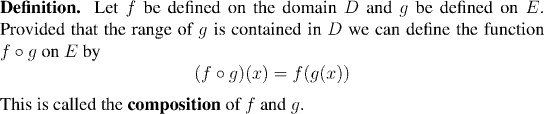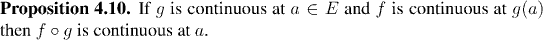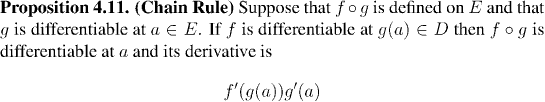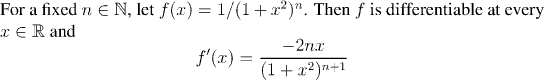Analysis WebNotes
arrow_back arrow_forward

# Class Contents

## Composition of FunctionsUsing these last few propositions we can prove many of the kinds of identities that one comes across as exercises in differentiation in calculus.

Example:## Where Are We Now?

Many of the results that we remember from calculus can be derived from the results we already have. The Chain Rule, the Product Rule and the Quotient Rule are the real mainstays of differentiation in calculus class, and we have these in their final forms. The problem we are still faced with, as mentioned at the end of the last class, is that we still don't know very much about some of the standard functions of analysis: the exponential functions and logarithms. Although as a result of the digression on exponential functions (and Homework 6) we now know quite a lot about the exponential and logarithmic functions, there are still huge gaps in our knowledge.

• We can't differentiate a^x or even x^b (unless b is an integer).
• Important rules from calculus are missing, such as f'(x) positive, implies f(x) is increasing. That means we can't make use of differentiation to find local maxima and minima yet.
We'll come back to these in the end of Chapter 6 and in Chapter 7.

The final section in this chapter deals with variations on the theme of limits of functions: left-hand and right-hand limits, and limits as x approaches infinity.